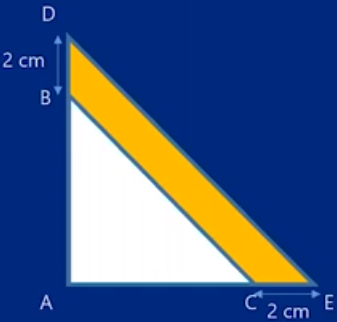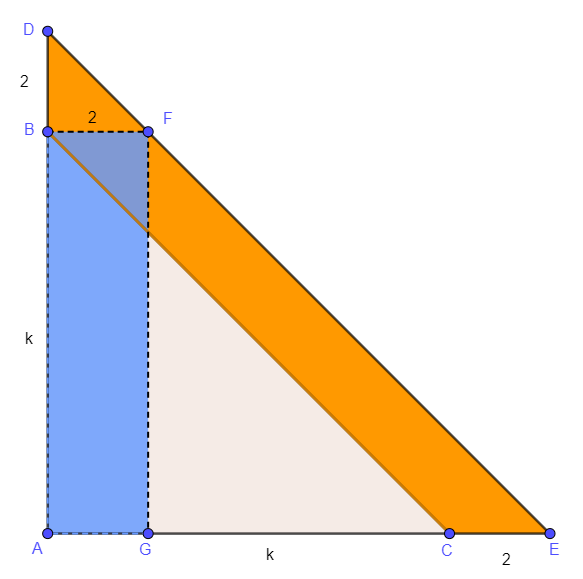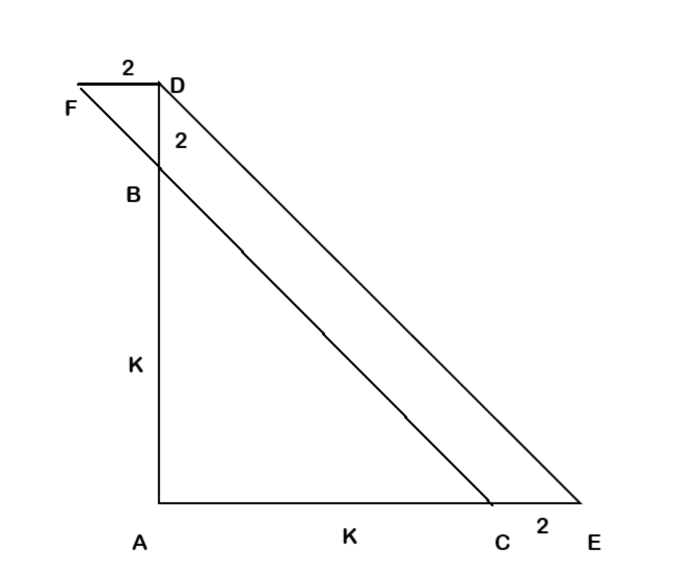# QuestionIn the figure, ABC and ADE are right-angled isosceles triangles. BD=CE=2cm. The area of the shaded part is 18cm². Find the length of AB.

I’m trying to find a method to solve this question other than guess and check. Can anyone help me?

```Solution 1:
Consider the blue rectangle BFGA.
It has the same height and width as parallelogram BFEC.
So they both have the same area, 2k.
The triangle DBF has area 2 x 2 x 0.5 = 2.
Orange strip has area
18 = DBF + BFEC = 2 + 2k,
which implies k = 8.
Therefore AB is 8cm.
Check: 0.5 x 10 x 10 - 0.5 x 8 x 8= 50 - 32 = 18``````Solution 2: (if you know algebra)
Area ADE = 0.5 x (k+2)**2 = 0.5 (k**2 + 2k + 4) = 0.5 k**2 + 2k + 2
Area ABC = 0.5 x k**2
So orange strip has area
18 = ADE - ABC = 0.5 k**2 + 2k + 2 - 0.5 x k**2 = 2k + 2,
which implies k = 8.
So AB is 8cm.

The equation is essentially the same 2k+2 = 8 in both solutions.
The algebra is interpreted as area in Solution 1.```Extend line BC and draw another horizontal line FD to form parallelogram CEFD as shown in diagram

Let AB=AC=k and

Area of shape BCDE = Area of Parallelogram CEFD –  Area of triangle FBD

18 = 2(k+2) – ½*2*2

18 = 2k +4 -2

2k = 16

K = 8

So length of AB is 8cm

0 Replies 1 Like